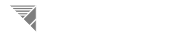The Simulator Formula Reference Page A Website For The AP Create Task# Step 1: Calculating The Wind Resistance Factors

#### Since a rocket begins it's flight on Earth's surface, it needs to pass through the atmoshpere in order to break away into low-earth orbit and beyond. Because of atmospheric influence, we have to consider the aerodynamics and flight parametrics involved with our rocket's passing of the atmosphere, considering multiple sub-variables such as the aerodynamics of the nosecone, the gravitational downforce, and the overall drag of the rocket. By utilizing the formula to calculate the wind resistance factors of a rocket, we can combine all of the listed disruptancies under a single vriable. By simply inputting the shape of the nosecone and the rocket's diameter, we can derive this variable that we'll use in the folowing calculations.

1) Please Select What Shape Nosecone You Would Like Your Rocket to Have:

Cone
Cube
Half Sphere

2) Now Enter the Desired Width of Your Preliminary Rocket. *NOTE - Only Integer Values Are Accepted

# Step 2: Calculating Velocity At Burnout

#### When a rocket's engine first ignites, it's propulsion begins to burn, eventually lowering to zero. The point in which the fuel runs out is known as the point of burnout and in order to calculate this, we first need to derive the velocity of the rocket at it's burnout point. To do this, you need to enter the thrust of your preliminary rocket so that it corresponds with it's weight. However, this is already calculated using the pre-derived thrust to weight ratio derived from the wind resistance factors you calculated in the previous program.

Mass in kg:

Thrust in kg:

# Step 3: Calculating Position of Burnout

#### With the burnout velocity derived, we can now calculate the point at which egnition stops, being the position of burnout. By using the thrust emmited by a rocket, the program pre-calcuates the thrust to burn time ratio as depicted with the slider's movement to clculate how far the rocket will travel.

Motor Burn Time: